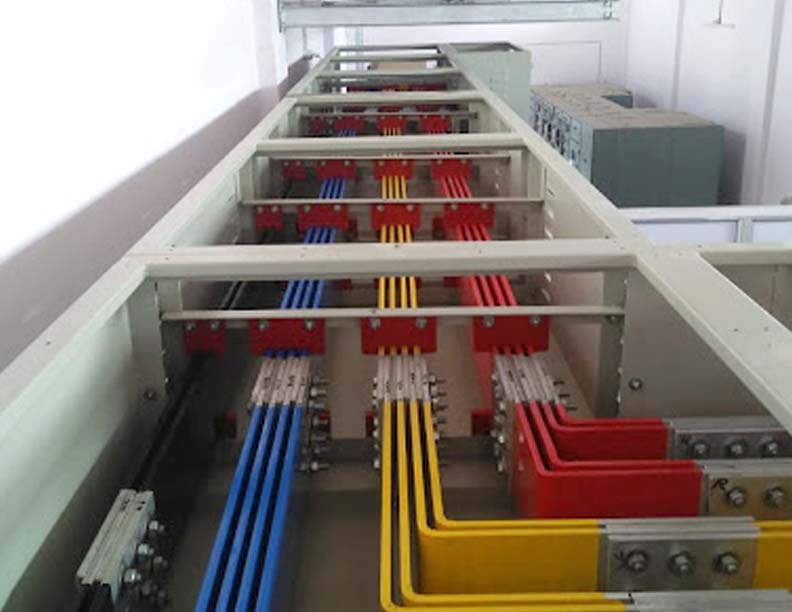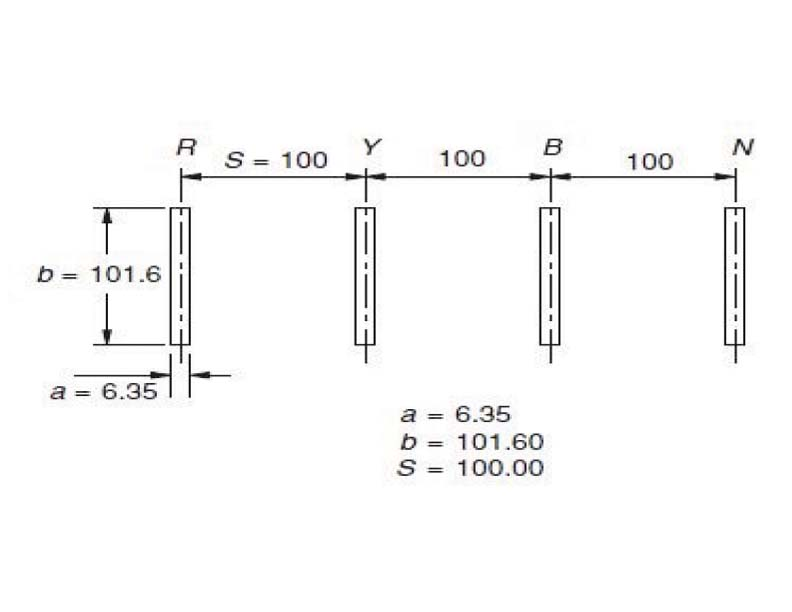# BUSDUCT SYSTEM DESIGN – Part 2

In order to have low losses and enhanced reliability in the distribution system, bus-ducts are preferred over cables for higher current ratings and shorter lengths at different voltage levels. Though Sandwich Bustrunking System with rated operational voltage (Ue) 1000 Volt are easily available, when it comes to economics–Air insulated bus ducts have an edge. This article focuses on the types of air insulated busducts and their selection guidelines, design requirements, de-rating factors and sizing requirement...Voltage Drop

The voltage drop across a bus system should be as low as possible and generally within 2% of the rated voltage. The length of the bus system in most of the applications may not be long enough so as to cause a voltage drop IZ. It may be the connection from the incoming transformer to the main receiving switchgear. Applications requiring long current carrying conductors, however, may have large impedance and may cause high voltage drops of the order of 3-5% and even more. When so, they may affect the stability of the system as well as the performance of the connected load. To fix the voltage drop in such cases it is essential to ascertain the actual values of the conductor’s own resistance, reactance and the impedance under actual operating conditions. It may be noted that reactance is the major cause of a high voltage drop. Skin and proximity effects play a vital role in affecting the resistance and reactance of such systems.

Skin effect and proximity effects on a current carrying conductor

In a DC system the current distribution through the cross section of a current carrying conductor is uniform as it consists of only the resistance. In an AC system the inductive effect caused by the induced electric field causes skin and proximity effects. These effects play a vital and complex role in determining the current distribution through the cross section of a conductor. In an AC system, the inductance of a conductor varies with respect to the depth of the conductor due to skin effect. This inductance is further affected by the presence of another current carrying conductor in the vicinity, which is known as proximity effect. Both these factors of an AC system tend to increase as the effective resistance and the impedance of the conductor cause a higher I2R loss and a higher voltage drop IZ and reduce its current carrying capacity.

Skin effect

A current carrying conductor produces an electric field

around it which induces a back emf and causes an inductive effect. This emf is produced in the conductor by its own electric field cutting the conductor. It is denser at the centre and less at the surface. The conductor thus has a higher inductance at the centre than at the surface and results in an uneven distribution of current through its own cross section. The current tends to concentrate at the outer surface of the conductor i.e. its skin shares more current than the other parts of the conductor and reduces with depth.

The phenomenon of uneven distribution of current within the same conductor due to the inductive effect is known as the skin effect and results in an increased effective resistance of the conductor. The ratio of AC to DC resistance, Rac/Rdc, is the measure of the skin effect and is known as the ‘skin effect ratio’. Figure-4 illustrates the skin effect for rectangular sections.

Determining the skin effect

Ratings of up to 3200A are normally required for distribution purposes such as for interconnecting a distribution transformer to a PCC, or a large PCC to another large PCC in a substation. The common practice for making such connections is to use rectangular cross sections, which are easy to handle, make joints compared to a channel or tubular section. It is however preferred to use such sections for ratings above 4000A for better utilization of active metal compared to flat sections.Example-2: Consider a section of 101.6mm x 6.35mm of

grade EIE-M as in figure-5, the resistance considering skin effect would be

Figure-5:

Rdc = 44.55 x 10-6ohm/m at 20deg.C (from table-12)

Area = 101.6 x 6.35 x 10-2 cm2 = 6.4516 cm2

Since the operating temperature should be considered to be 85deg.C

Rdc at 85deg.C = Rdc 20 (1+α2021))

α20= temperature coefficient of resistance for Aluminium = 0.00403 at 20deg.C

Rdc = 44.55(1+0.00403(85-20) =0.056 ohm/1000m

Now refer to figure-4 to obtain the skin effect ratio Rac/Rdc. Consider the cross-sectional curves for EIE-M grade of flat busbars at an operating temperature of 85 deg.C for a cross sectional area of 6.45 cm2 and the Rac/Rdc ratio on the skin effect curve would be

b/a = 101.6/6.35 = 16

Rac/Rdc = 1.055

i.e. an increase of almost 5.5% due to the skin effect alone and Rac = 1.055 x 0.056 = 0.059 ohm/1000mSkin effect for more than one conductor per phase

In such cases, the group of busbars in each phase may be considered to be one large conductor and outside dimensions a and b as illustrated in figure-6 measured for all calculations.

Example-3:

Considering a four conductor system of section 101.6mm x 6.35mm in each phase (Figure-7) of grade ELE-M for a

current of 2000A, Rac would be

As calculate before

Rdc = 0.056 ohm/ 1000m per conductor of four bus sections in parallel

Skin effect ratio from graph at an operating temperature of 85 deg.C for a cross sectional area of 25.8cm2 for an EIE-M grade of Aluminium having

b/a = 101.6/44.45 = 2.3

Rac/Rdc ≈ 1.33

Rac for the phase = 1.33 x 0.056 / 4 = 18.62 x 10-3 ohm

/1000m (as four sections in parallel)

Proximity Effect

If there exists more than one current carrying conductor not of the same phase, placed adjacent to each other, so that the electric field produced by one can link the other and thus mutual induction will take place. The magnitude of this will depend upon the amount of current and the spacing between the two. This tends to further distort the self-resistance of the conductor in addition to the distortion already caused by the skin effect current distribution through its cross section. Figure-8 shows distortion of current flow in a round conductor and also the mechanical forces exerted on the conductors, due to this distorted current distribution. There is always a force between two current carrying conductors placed adjacent to each other, be it DC or an AC system. The proximity effect, however is limited to an AC system due to mutual induction between the two current carrying conductors.

The displacement of current and the forces on the conductors are two different effects. The effect of current displacement is to increase the effective resistance and the impedance of the conductor on one side as shown in figure-8 (b) & (c) and cause a distortion in its heating pattern. This will lead the various conductors of a particular phase to operate at different temperatures and contribute to I2R losses. The rating of all the conductors of one phase must therefore be determined by the hottest conductor. The distortion of current will also distort the heat produced. The area having high current density will produce higher heat. The proximity effect thus also causes a derating in the current carrying capacity of a conductor.

The proximity effect is directly proportional to the magnitude of the current and inversely proportional to the spacing between the two conductors as stated earlier. The smaller the phase spacing, the greater will be the effect of proximity as well as derating and vice versa. But the reactance of the two phases is directly proportional to the spacing. Reactance is the main cause of an excessive voltage drop. The smaller the spacing, the lower will be the reactance and vice versa. While the requirement of a lower reactance will require less spacing and will mean higher forces, demanding stronger busbar supports and mounting structure. Contrarily, requiring a lower effect on current carrying capacity would require a larger spacing between the phases, which would result in a higher reactance and consequently a higher voltage drop. But a high reactance would help to reduce the level of fault current and also forces between conductors.

A compromise is therefore required to meet both the needs and obtain a more balanced system. In that case, other methods can also be adopted to reduce the skin and proximity effects.

Proximity effect in terms of busbar reactance

Reactance Xa of the conductor plays a significant role in transmitting the power through a bus system from one end to other. For long bus systems, it must be determined at the design stage whether the voltage drop in the total bus length on account of this will fall within the permissible limits, particularly for higher ratings (2000A and above) besides the current carrying capacity. A higher reactance means higher drop. For smaller ratings and shorter lengths, as well as HT systems, this drop would be too low as a percentage of the rated voltage. For higher ratings, however it may assume a greater significance and precautionary measures may become necessary to restrict it within permissible limits. To determine Xa, proximity effect curves have been established by conducting tests on the metal and are available for rectangular section. Figure-9 shows the curve for rectangular section for 50Hz.

The reactance is drawn as a function of Centre spacing/ Semi-perimeter (a+b). At lower spacings this value will be influenced by the width and thickness of the conductor. At lower spacings, therefore proximity curves are different for different ratios of a/b whereas for large spacings they approach the same curve. When more than one section is used together, to make larger ratings, all the sections of one phase may be considered to be one large section.Chanukya Annepu

The author is from the Engineering Services Division, Indira Gandhi Centre for Atomic Research, Kalpakkam, India